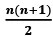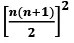# Important Formulas of Arithmetic Progression and Geometric Progression

1. Arithmetic Progression Formulae

• an = a1 + (n – 1)d• Number of terms =Sum of first n natural numbers
⇒ 1 + 2 + 3 … + n =Sum of squares of first n natural numbers
⇒ 12 + 22 + 32 + … + n2 =Sum of cubes of first n natural numbers
⇒ 13 + 23 + 33 … + n3 =• Sum of first n odd numbers
⇒ 1 + 3 + 5 … + (2n – 1) = n2
• Sum of first n even numbers
⇒ 2 + 4 + 6 … 2n = n(n – 1)
• If you have to consider 3 terms in an AP, consider {a-d, a, a+d}. If you have to consider 4 terms, consider {a-3d, a-d, a+d, a+3d}
• If all terms of an AP are multiplied with k or divided with k, the resultant series will also be an AP with the common difference dk or d/k respectively.

2. Geometric Progression Formulae

The list of formulas related to GP is given below which will help in solving different types of problems.

• The general form of terms of a GP is a, ar, ar2, ar3, and so on. Here, a is the first term and r is the common ratio.
• The nth term of a GP is Tn = arn-1
• Common ratio = r = Tn/ Tn-1
• The formula to calculate the sum of the first n terms of a GP is given by:
Sn = a[(r– 1)/(r – 1)] if r ≠ 1and r > 1
Sn = a[(1 – rn)/(1 – r)] if r ≠ 1 and r < 1
• The nth term from the end of the GP with the last term l and common ratio r = l/ [r(n – 1)].
• The sum of infinite, i.e. the sum of a GP with infinite terms is S= a/(1 – r) such that 0 < r < 1.
• If three quantities are in GP, then the middle one is called the geometric mean of the other two terms.
• If a, b and c are three quantities in GP, then and b is the geometric mean of a and c. This can be written as b2 = ac or b =√ac
• Suppose a and r be the first term and common ratio respectively of a finite GP with n terms. Thus, the kth term from the end of the GP will be = arn-k.
Scroll to Top